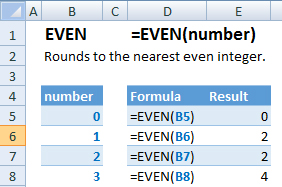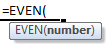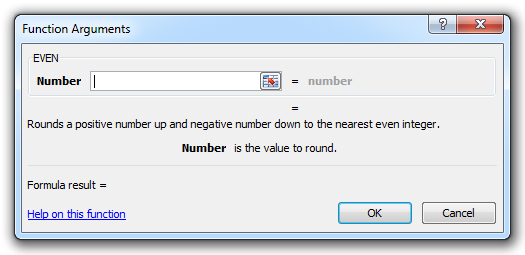### EVEN Function Examples – Excel, VBA, & Google Sheets

This Excel Tutorial demonstrates how to use the Excel EVEN Function in Excel to round to the nearest even integer, with formula examples.

## EVEN Function Description:

The EVEN Function Rounds to the nearest even integer. Positive numbers round up. Negative numbers round down.

## Formula Examples:Example Formula Result
1 =EVEN(B5) 0
2 =EVEN(B6) 2
3 =EVEN(B7) 2
4 =EVEN(B8) 4
AutoMacro - VBA Code Generator

## Syntax and Arguments:

The Syntax for the EVEN Formula is:

`=EVEN(number)`

### Function Arguments ( Inputs ):

number – A number.

## Additional Notes

Use the EVEN Function to round a number to the nearest even integer. Simply enter any number directly into the formula, or reference a cell containing a number.

## EVEN Examples in VBA

You can also use the EVEN function in VBA. Type:
`application.worksheetfunction.even(number)`
For the function arguments (number, etc.), you can either enter them directly into the function, or define variables to use instead.

Return to the List of all Functions in Excel

## How to use the EVEN Function in Excel:

To use the AND Excel Worksheet Function, type the following into a cell:
`=AND(`
After entering it in the cell, notice how the AND formula inputs appear below the cell:You will need to enter these inputs into the function. The function inputs are covered in more detail in the next section. However, if you ever need more help with the function, after typing “=EVEN(” into a cell, without leaving the cell, use the shortcut CTRL + A (A for Arguments) to open the “Insert Function Dialog Box” for detailed instructions:For more information about the EVEN Formula visit the
Microsoft Website.

## Excel Practice Worksheet

Practice Excel functions and formulas with our 100% free practice worksheets!

• Automatically Graded Exercises
• Learn Excel, Inside Excel!